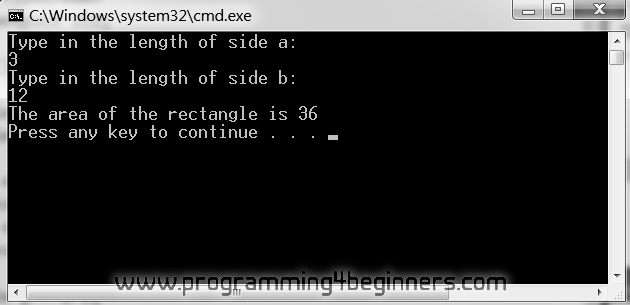## 4. Variables

### Reading Data from Standard Input

Look at this program for computing the area of a rectangle, given the lengths of its sides a and b:

```#include <iostream>
using namespace std;

int main()
{
double a = 4;
double b = 5;
double A = a*b;

cout << "The area of the rectangle is " << A << endl;
}
```

When you execute it, you should get the following in the console window:

```The area of the rectangle is 20
```

What if we now wanted to compute the area of a different rectangle? We would have to rewrite and potentially recompile this program (if we intend to execute it using a computer). Although, at this stage, you might not see this as problematic, there is a way of writing a single program that would compute the area of any rectangle, given the lengths of its sides.

For that to work, our programs need to receive data from the outside world. In this case, we can imagine a person typing in the required data. This can be achieved by using standard input. The following program demonstrates this:

```#include <iostream>
using namespace std;

int main()
{
double a = 0;
double b = 0;

cout << "Type in the length of side a:" << endl;
cin >> a;
cout << "Type in the length of side b:" << endl;
cin >> b;

double A = a*b;

cout << "The area of the rectangle is " << A << endl;
}
```

Type this program in your IDE and execute it using Debug -> Start Without Debugging (or simply press the Ctrl-F5 key combo). The program will ask you to enter the sides of a rectangle of which the area is to be computed. Type in a number and press Enter. Do this one more time. After you have entered the lengths of both sides, the area of the rectangle will be written out:The novelty in this program is a new word: `cin`. In our programs, `cin` represents standard input, short for character input, as `cout` is short for character output. By default, when a computer executes a program, standard input will come from the keyboard.

Notice how, in statements beginning with the word `cin` (from now on we will just call them "`cin` statements") the symbols '`>>`' point in the opposite direction of those in `cout` statements. In `cin` statements, the symbols '`>>`' always point towards, or into, the variable, because variables are used as a destination for values extracted from standard input. It is the opposite with `cout` statements: the symbols '`<<`' point away from the expression and towards `cout`, since standard output is a destination for the provided values.

When executing a `cin` statement, a value read from standard input is stored in the variable specified immediately after the symbols '`>>`'. When used in this manner, the symbols '`>>`' are called the extraction operator. Similarly, and just to remind you, the symbols '`<<`' are called the insertion operator.

A person using our program and typing in the required input data is called a user. The data typed into our program by the user, or provided to the program by any other means, is called the input data. For the program above we imagine that a user will type in the lengths of sides of a rectangle as the input data.